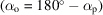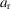International Tables for Crystallography (2010). Vol. B, ch. 4.6, pp. 590-624   | 1 | 2 | https://doi.org/10.1107/97809553602060000778

## Contents

• 4.6. Reciprocal-space images of aperiodic crystals  (pp. 590-624)
• 4.6.1. Introduction  (pp. 590-591) | html | pdf |
• 4.6.2. The n -dimensional description of aperiodic crystals  (pp. 591-598) | html | pdf |
• 4.6.2.1. Basic concepts  (p. 591) | html | pdf |
• 4.6.2.2. 1D incommensurately modulated structures  (pp. 591-593) | html | pdf |
• 4.6.2.3. 1D composite structures  (pp. 593-594) | html | pdf |
• 4.6.2.4. 1D quasiperiodic structures  (pp. 594-597) | html | pdf |
• 4.6.2.5. 1D structures with fractal atomic surfaces  (pp. 597-598) | html | pdf |
• 4.6.3. Reciprocal-space images  (pp. 598-621) | html | pdf |
• 4.6.3.1. Incommensurately modulated structures (IMSs)  (pp. 598-602) | html | pdf |
• 4.6.3.1.1. Indexing  (p. 599) | html | pdf |
• 4.6.3.1.2. Diffraction symmetry  (pp. 599-600) | html | pdf |
• 4.6.3.1.3. Structure factor  (pp. 600-602) | html | pdf |
• 4.6.3.2. Composite structures (CSs)  (pp. 602-603) | html | pdf |
• 4.6.3.2.1. Indexing  (p. 603) | html | pdf |
• 4.6.3.2.2. Diffraction symmetry  (p. 603) | html | pdf |
• 4.6.3.2.3. Structure factor  (p. 603) | html | pdf |
• 4.6.3.3. Quasiperiodic structures (QSs)  (pp. 603-621) | html | pdf |
• 4.6.3.3.1. 3D structures with 1D quasiperiodic order  (pp. 603-607) | html | pdf |
• 4.6.3.3.1.1. Indexing  (pp. 603-604) | html | pdf |
• 4.6.3.3.1.2. Diffraction symmetry  (p. 604) | html | pdf |
• 4.6.3.3.1.3. Structure factor  (pp. 604-605) | html | pdf |
• 4.6.3.3.1.4. Intensity statistics  (pp. 605-606) | html | pdf |
• 4.6.3.3.1.5. Relationships between structure factors at symmetry-related points of the Fourier image  (pp. 606-607) | html | pdf |
• 4.6.3.3.2. Decagonal phases  (pp. 607-613) | html | pdf |
• 4.6.3.3.2.1. Indexing  (p. 610) | html | pdf |
• 4.6.3.3.2.2. Diffraction symmetry  (pp. 610-611) | html | pdf |
• 4.6.3.3.2.3. Structure factor  (pp. 611-612) | html | pdf |
• 4.6.3.3.2.4. Intensity statistics  (pp. 612-613) | html | pdf |
• 4.6.3.3.2.5. Relationships between structure factors at symmetry-related points of the Fourier image  (p. 613) | html | pdf |
• 4.6.3.3.3. Icosahedral phases  (pp. 613-621) | html | pdf |
• 4.6.3.3.3.1. Indexing  (pp. 616-618) | html | pdf |
• 4.6.3.3.3.2. Diffraction symmetry  (p. 618) | html | pdf |
• 4.6.3.3.3.3. Structure factor  (p. 619) | html | pdf |
• 4.6.3.3.3.4. Intensity statistics  (p. 619) | html | pdf |
• 4.6.3.3.3.5. Relationships between structure factors at symmetry-related points of the Fourier image  (pp. 619-621) | html | pdf |
• 4.6.4. Experimental aspects of the reciprocal-space analysis of aperiodic crystals  (pp. 621-623) | html | pdf |
• 4.6.4.1. Data-collection strategies  (pp. 621-623) | html | pdf |
• 4.6.4.2. Commensurability versus incommensurability  (p. 623) | html | pdf |
• 4.6.4.3. Twinning and nanodomain structures  (p. 623) | html | pdf |
• References | html | pdf |
• Figures
• Fig. 4.6.2.1. The combination of a basic structure, with period a , and a sinusoidal modulation function, with amplitude A , period λ and, gives a modulated structure (MS)(p. 591) | html | pdf |
• Fig. 4.6.2.2. 2D embedding of the sinusoidally modulated structure illustrated in Fig. 4.6.2.1  (p. 592) | html | pdf |
• Fig. 4.6.2.3. Schematic representation of the 2D reciprocal-space embedding of the 1D sinusoidally modulated structure depicted in Figs. 4.6.2.1 and 4.6.2.2  (p. 593) | html | pdf |
• Fig. 4.6.2.4. 2D embedding of a 1D composite structure without mutual interaction of the subsystems  (p. 593) | html | pdf |
• Fig. 4.6.2.5. 2D embedding of a 1D composite structure with mutual interaction of the subsystems causing modulations  (p. 594) | html | pdf |
• Fig. 4.6.2.6. Schematic representation of the reciprocal space of the embedded 1D composite structure depicted in Fig. 4.6.2.4  (p. 594) | html | pdf |
• Fig. 4.6.2.7. Schematic representation of the reciprocal space of the embedded 1D composite structure depicted in Fig. 4.6.2.5  (p. 594) | html | pdf |
• Fig. 4.6.2.8. 2D embedding of the Fibonacci chain  (p. 596) | html | pdf |
• Fig. 4.6.2.9. Schematic representation of the reciprocal space of the embedded Fibonacci chain depicted in Fig. 4.6.2.8  (p. 596) | html | pdf |
• Fig. 4.6.2.10. Reciprocal space of the embedded Fibonacci chain as a modulated structure  (p. 597) | html | pdf |
• Fig. 4.6.2.11. 2D direct-space embedding of the Fibonacci chain as a modulated structure  (p. 598) | html | pdf |
• Fig. 4.6.2.12. ( a ) Three steps in the development of the fractal atomic surface of the squared Fibonacci sequence starting from an initiator and a generator  (p. 598) | html | pdf |
• Fig. 4.6.3.1. Schematic diffraction patterns for IMSs with ( a ) 1D, ( b ) 2D and ( c ) 3D modulation  (p. 599) | html | pdf |
• Fig. 4.6.3.2. The relationships between the coordinatesand the modulation functionin a special section of thespace  (p. 601) | html | pdf |
• Fig. 4.6.3.3. Schematic diffraction patterns for 3D IMSs with ( a ) 1D harmonic and ( b ) rectangular density modulation  (p. 602) | html | pdf |
• Fig. 4.6.3.4. The relative structure-factor magnitudes of m th-order satellite reflections for a harmonic displacive modulation are proportional to the values of the m th-order Bessel function(p. 602) | html | pdf |
• Fig. 4.6.3.5. The structure factors(below) and their magnitudes(above) of a Fibonacci chain decorated with equal point atoms are shown as a function of the parallel-space componentof the diffraction vector  (p. 604) | html | pdf |
• Fig. 4.6.3.6. The structure factors(below) and their magnitudes(above) of a Fibonacci chain decorated with equal point atoms are shown as a function of the perpendicular-space componentof the diffraction vector  (p. 604) | html | pdf |
• Fig. 4.6.3.7. The structure factorsof the Fibonacci chain decorated with aluminium atoms ( U overall = 0.005 Å 2 ) as a function of the parallel (above) and the perpendicular (below) component of the diffraction vector  (p. 605) | html | pdf |
• Fig. 4.6.3.8. The structure factors(below) and their magnitudes(above) of the squared Fibonacci chain decorated with equal point atoms are shown as a function of the parallel-space componentof the diffraction vector  (p. 606) | html | pdf |
• Fig. 4.6.3.9. The structure factors(below) and their magnitudes(above) of the squared Fibonacci chain decorated with equal point atoms are shown as a function of the perpendicular-space componentof the diffraction vector  (p. 606) | html | pdf |
• Fig. 4.6.3.10. Part … LSLLSLSL … of a Fibonacci sequencebefore and after scaling by the factor τ  (p. 607) | html | pdf |
• Fig. 4.6.3.11. Scaling operations of the Fibonacci sequence  (p. 607) | html | pdf |
• Fig. 4.6.3.12. Reciprocal basis of the decagonal phase in the 5D description projected upon(above left) and(above right)  (p. 608) | html | pdf |
• Fig. 4.6.3.13. A section of a Penrose tiling (thin lines) superposed by its τ-deflated tiling (above, thick lines) and by its-deflated tiling (below, thick lines)  (p. 609) | html | pdf |
• Fig. 4.6.3.14. Scaling symmetry of a pentagram superposed on the Penrose tiling  (p. 609) | html | pdf |
• Fig. 4.6.3.15. Atomic surface of the Penrose tiling in the 5D hypercubic description  (p. 610) | html | pdf |
• Fig. 4.6.3.16. Projection of the 4D hyper-rhombohedral unit cell of the Penrose tiling in the 4D description upon the perpendicular space  (p. 610) | html | pdf |
• Fig. 4.6.3.17. Schematic diffraction pattern of the Penrose tiling (edge length of the Penrose unit rhombs a r = 4.04 Å)  (p. 611) | html | pdf |
• Fig. 4.6.3.18. Enlarged section of Fig. 4.6.3.17  (p. 611) | html | pdf |
• Fig. 4.6.3.19. The perpendicular-space diffraction pattern of the Penrose tiling (edge length of the Penrose unit rhombs a r = 4.04 Å)  (p. 611) | html | pdf |
• Fig. 4.6.3.20. Enlarged section of Fig. 4.6.3.19 showing the projected 4D reciprocal-lattice unit cell  (p. 611) | html | pdf |
• Fig. 4.6.3.21. Radial distribution function of the structure factorsof the Penrose tiling (edge length of the Penrose unit rhombs a r = 4.04 Å) decorated with point atoms as a function of(p. 612) | html | pdf |
• Fig. 4.6.3.22. Radial distribution function of the structure factorsof the Penrose tiling (edge length of the Penrose unit rhombs a r = 4.04 Å) decorated with point atoms as a function of(p. 613) | html | pdf |
• Fig. 4.6.3.23. Radial distribution function of the structure-factor magnitudesof the Penrose tiling (edge length of the Penrose unit rhombs a r = 4.04 Å) decorated with point atoms as a function of(p. 613) | html | pdf |
• Fig. 4.6.3.24. Pentagrammal relationships between scaling symmetry-related positive structure factorsof the Penrose tiling (edge length a r = 4.04 Å) in parallel space  (p. 614) | html | pdf |
• Fig. 4.6.3.25. Pentagrammal relationships between scaling symmetry-related negative structure factorsof the Penrose tiling (edge length a r = 4.04 Å) in parallel space  (p. 614) | html | pdf |
• Fig. 4.6.3.26. Pentagrammal relationships between scaling symmetry-related structure factorsof the Penrose tiling (edge length a r = 4.04 Å) in parallel space  (p. 614) | html | pdf |
• Fig. 4.6.3.27. Pentagrammal relationships between scaling symmetry-related structure factorsof the Penrose tiling (edge length a r = 4.04 Å) in perpendicular space  (p. 614) | html | pdf |
• Fig. 4.6.3.28. Perspective ( a ) parallel- and ( b ) perpendicular-space views of the reciprocal basis of the 3D Penrose tiling  (p. 615) | html | pdf |
• Fig. 4.6.3.29. Schematic representation of a rotation in 6D space  (p. 615) | html | pdf |
• Fig. 4.6.3.30. The two unit tiles of the 3D Penrose tiling: a prolateand an oblaterhombo­hedron with equal edge lengths(p. 616) | html | pdf |
• Fig. 4.6.3.31. Atomic surface of the 3D Penrose tiling in the 6D hypercubic description  (p. 616) | html | pdf |
• Fig. 4.6.3.32. Perspective parallel-space view of the two alternative reciprocal bases of the 3D Penrose tiling  (p. 616) | html | pdf |
• Fig. 4.6.3.33. Physical-space diffraction patterns of the 3D Penrose tiling decorated with point atoms  (p. 617) | html | pdf |
• Fig. 4.6.3.34. Perpendicular-space diffraction patterns of the 3D Penrose tiling decorated with point atoms  (p. 618) | html | pdf |
• Fig. 4.6.3.35. Radial distribution function of the structure factorsof the 3D Penrose tiling  (p. 619) | html | pdf |
• Fig. 4.6.3.36. Parallel-space distribution of ( a ) positive and ( b ) negative structure factors of the 3D Penrose tiling of the 6D P lattice type decorated with point atoms  (p. 620) | html | pdf |
• Fig. 4.6.3.37. Perpendicular-space distribution of ( a ) positive and ( b ) negative structure factors of the 3D Penrose tiling of the 6D P lattice type decorated with point atoms  (p. 620) | html | pdf |
• Fig. 4.6.4.1. Simulated diffraction patterns of ( a ) thesingle-crystal approximant of decagonal Al–Co–Ni, ( b ) the fivefold twinned approximant, and ( c ) the decagonal phase itself  (p. 621) | html | pdf |
• Fig. 4.6.4.2. Zero-layer X-ray diffraction patterns of decaprismatic Al 73.5 C 21.7 Ni 4.8 crystals  (p. 622) | html | pdf |
• Tables
• Table 4.6.2.1. Expansion of the Fibonacci sequenceby repeated action of the substitution rule σ:,(p. 595) | html | pdf |
• Table 4.6.3.1. 3D point groups of order k describing the diffraction symmetry and corresponding 5D decagonal space groups with reflection conditions (see Rabson et al., 1991)  (p. 612) | html | pdf |
• Table 4.6.3.2. 3D point groups of order k describing the diffraction symmetry and corresponding 6D decagonal space groups with reflection conditions (see Levitov & Rhyner, 1988; Rokhsar et al., 1988)  (p. 618) | html | pdf |
• Table 4.6.4.1. Intensity statistics of the Fibonacci chain for a total of 161 322 reflections withand(p. 620) | html | pdf |